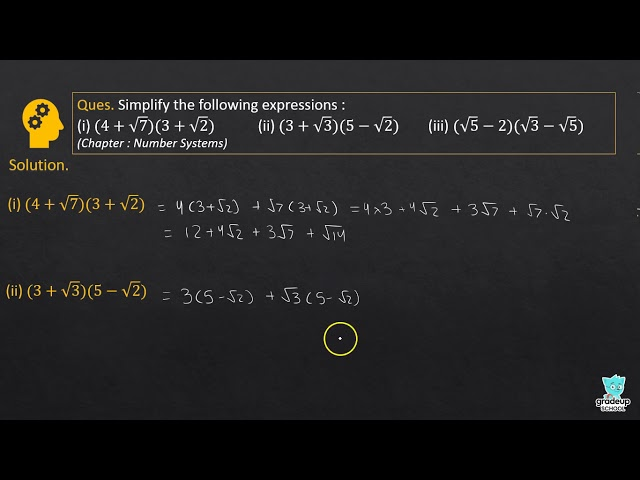# RD Sharma Class 9 Maths Solutions Chapter 3 - Rationalisation

Share

## RD Sharma Class 9 Maths Solutions Chapter 3 - Rationalisation

Exercise 3.1
• Exercise 3.1
• Exercise 3.2
• CCE - Formative Assessment
Class 9th|RD Sharma - MathematicsChapter 3 - RationalisationRD Sharma - MathematicsClass 9th , RD Sharma
 Chapter 1 - Number System Chapter 2 - Exponents of Real Numbers Chapter 3 - Rationalisation Chapter 4 - Algebraic Identities Chapter 5 - Factorization of Algebraic Expressions Chapter 6 - Factorization of Polynomials Chapter 7 - Introduction to Euclid's Geometry Chapter 8 - Lines and Angles Chapter 9 - Triangle and Its Angles Chapter 10 - Congruent Triangles Chapter 11 - Co-ordinate Geometry Chapter 13 - Linear Equations in Two Variables Chapter 12 - Heron's Formula Chapter 14 - Quadrilaterals Chapter 15 - Areas of Parallelograms Chapter 16 - Circles Chapter 17 - Constructions Chapter 18 - Surface Area and Volume of a Cuboid and a Cude Chapter 19 - Surface Area and Volume of a Right Circular Cylinder Chapter 20 - Surface Area and Volume of a Right Circular Cone Chapter 21 - Surface Area and Volume of a Sphere Chapter 22 - Tabular Representation of Statistical Data Chapter 23 - Graphical Representation of Statistical Data Chapter 24 - Measures of Central Tendency Chapter 25 - Probability